Courses

# Ex 14.4 NCERT Solutions- Statistics Class 10 Notes | EduRev

## Class 10 : Ex 14.4 NCERT Solutions- Statistics Class 10 Notes | EduRev

The document Ex 14.4 NCERT Solutions- Statistics Class 10 Notes | EduRev is a part of the Class 10 Course Mathematics (Maths) Class 10.
All you need of Class 10 at this link: Class 10

NCERT TEXTBOOK QUESTIONS SOLVED
Page No. 293
EXERCISE 14.4
Q 1. The following distribution gives the daily income of 50 workers of a factory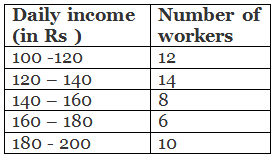Convert the distribution above to a less than type cumulative frequency distribution, and draw its ogive.
Sol. We have the cumulative frequency distribution as:

 Daily income (in Rs ) Cumulative frequencies Less than 120 12 + 0 = 12 Less than 140 12 + 14 = 26 Less than 160 26 + 8 = 34 Less than 180 34 + 6 = 40 Less than 200 40 + 10 = 50

Now, we plot the points corresponding to the ordered pairs (120, 12), (140, 26), (160, 34), (180, 40) and (200, 50) on a graph paper and join them by a free hand smooth curve as shown below: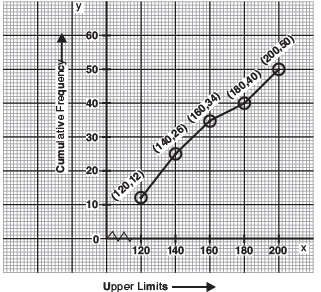The curve so obtained is called the less than o give.

Q 2. During the medical check-up of 35 students of a class, their weights were recorded as follows:

 Weight (in kg) Number of students Less than 38 0 Less than 40 3 Less than 42 5 Less than 44 9 Less than 46 14 Less than 48 28 Less than 50 32 Less than 52 35

Draw a less than type ogive for the given data. Hence obtain the median weight from the graph and verify the result by using the formula
Sol. Here, the values 38, 40, 42, 44, 46, 48, 50 and 52 are the upper limits of the respective class intervals.
We plot the points (ordered pairs) (38, 0), (40, 3), (42, 5), (44, 9), (46, 14), (48, 28), (50, 32) and (52, 35) on a graph paper and join them by a free hand smooth curve.
The curve so obtained is the less than type ogive.
∵ n = 35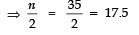The point 17.5 is on y-axis.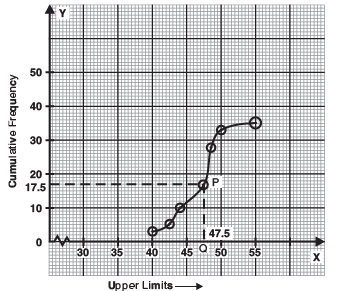From this point (i.e., from 17.5) we draw a line parallel to the x-axis which cuts the curve at P. From this point (i.e., from P), draw a perpendicular to the x-axis, meeting the x-axis at Q. The point Q represents the median of the data which is 47.5.
Verification
To verify the result using the formula, let us make the following table in order to find median using the formula:

 Weight (in kg) Number of students [Cumulative frequency] 0-38 0 38-40 3 40-42 5 42-44 9 44-46 14 46-48 28 48-50 32 50-52 35

Here,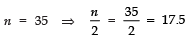Since, this observation lies in the class 46 − 48.
∴ The median class is 46 − 48 such that l = 46, h = 2, f = 14, cf = 14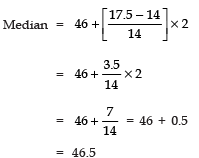Thus, the median = 46.5 kg is approximately verified.

Q 3. The following table gives production yield per hectare of wheat of 100 farms of a village.

 Production yield (in kg/ha) 50-55 55-60 60-65 65-70 70-75 75-80 Number of farms 2 8 12 24 38 16

Change the distribution to a more than type distribution, and draw its ogive.
Sol. For ‘more than type’ distribution, we have:

 Production yield (in kg/ha) Number of farms (Cumulative frequencies) More than 50 100 More than 55 98 More than 60 90 More than 65 78 More than 70 54 More than 75 16

Now, we plot the points (50, 100), (55, 98), (60, 90), (65, 78), (70, 54) and (75, 16) and join the points with a free hand curve.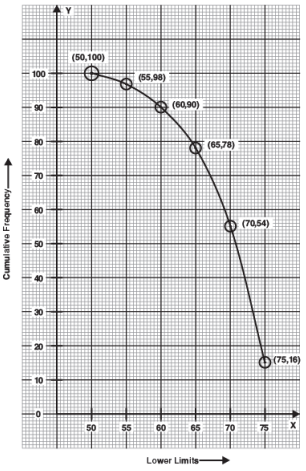The curve so obtained is the ‘more than type ogive’.

Offer running on EduRev: Apply code STAYHOME200 to get INR 200 off on our premium plan EduRev Infinity!

,

,

,

,

,

,

,

,

,

,

,

,

,

,

,

,

,

,

,

,

,

;